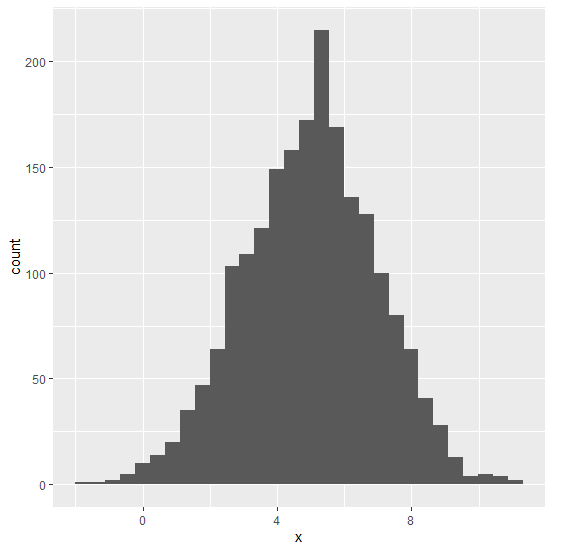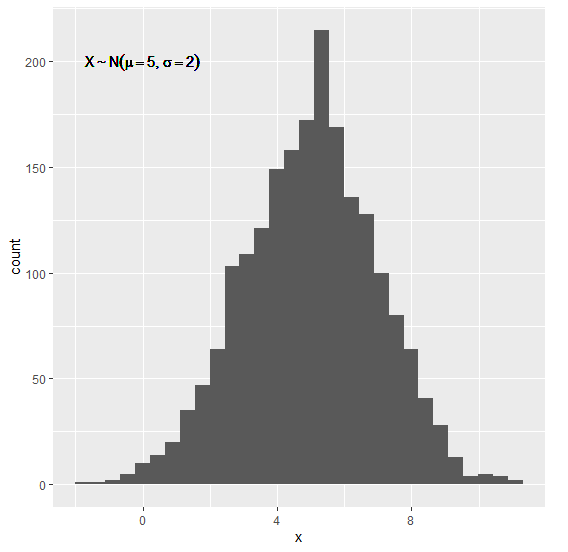# How to display tilde ggplot2 graph in R?

Let’s say we want to display tilde sign at a particular position in histogram using ggplot2 graph. In this situation, we can use geom_text function and pass all the text with label argument inside aes where tilde will be written as %~%.

For Example, if we want to display X follows Normal Distribution then we can write it as −

geom_text(aes(label="X %~% Normal Distribution",x=0,y=200),parse=TRUE)

Here, x=0 and y=200 is the position of the label in the histogram.

## Example

Consider the below data frame −

x<-rnorm(2000,5,2)
df<-data.frame(x)
head(df,20)

The following dataframe is created

         x
1 3.429893
2 2.873259
3 4.621022
4 4.873246
5 4.707371
6 7.650049
7 6.402318
8 4.427331
9 6.830767
10 4.535618
11 6.622828
12 4.770918
13 1.018385
14 5.725048
15 2.238412
16 6.623515
17 4.835721
18 5.266638
19 5.380624
20 4.381922

To load the ggplot2 package and create histogram of x on the above created data frame, add the following code to the above snippet −

x<-rnorm(2000,5,2)
df<-data.frame(x)
library(ggplot2)
ggplot(df,aes(x))+geom_histogram(bins=30)

## Output

If you execute all the above given snippets as a single program, it generates the following Output −To create a histogram of x with representing tilde sign in the graph on the above created data frame, add the following code to the above snippet −

x<-rnorm(2000,5,2)
df<-data.frame(x)
library(ggplot2)
ggplot(df,aes(x))+geom_histogram(bins=30)+geom_text(aes(label="X %~%
N(mu==5,sigma==2)",x=0,y=200),parse=TRUE)

## Output

If you execute all the above given snippets as a single program, it generates the following Output −